# 1st PUC Chemistry Question Bank Chapter 6 Thermodynamics

## Karnataka 1st PUC Chemistry Question Bank Chapter 6 Thermodynamics

### 1st PUC Chemistry Thermodynamics One Mark Questions and Answers

Question 1.
What is thermodynamics ?
Thermodynamics is a branch of chemistry that deals with the study of interconversion of with other forms energy during physical and chemical changes.

Question 2.
What you mean by enthalpy?
The total heat content of a system is called enthalpy.

Question 3.
Write the relationship between ∆H and ∆E.
∆H = ∆E + RT∆n, ∆H = Enthalpy change, ∆E = Internal energy change
∆n = Number of gaseous product number of gaseous reactant or number of moles.

Question 4.
Define (Heat of reaction) Enthalpy of reaction.
Heat of a reaction is the change in enthalpy produced when the number of moles of the reactants as represented in the balanced chemical equation have completely reacted with each other.

Question 5.
Heat (enthalpy) of formation.
Heat of formation of a compound is the change in enthalpy produced when one mole of the compound is formed from its elements denoted as ∆Hf.Question 6.
What is Heat Capacity ?
Het capacity of a system is defined as quantity of heat required to raise temperature by 1°.

Question 7.
What is specific heat capacity ?
It is defined as the quantity of heat required to raise temperature of 1 gram of a substance by 1° C or 1K

Question 8.
What is the relation between CP and Cv for an ideal gas.
CP– Cv = R

Question 9.
What is entropy ?
A measure of degree of disorder of a system.

Question 10.
What is the unit of entropy ?
J / K (Joule per Kelvin)

Question 11.
What is Gibbs-Helmholtz equation ?
∆G° = ∆H° – T∆S°.Question 12.
Give an expression for the work done in a reversible isothermal expansion of an ideal gas.
w = -2.303nRT log $$\frac{V_{2}}{V_{1}}$$

Question 13.
Explain : Heat of formation a acetylene is + 54 kcal.
When 1 mole of gaseous acetalylene is formed from its elements, carbon (s) and hydrogen (g), 54 kcal of heat is absorbed.

Question 14.
White phosphorous is less stable than red phosphorous. Mention whether the process of conversion of white phosphorous to red phosphorous is exothermic or endothermic reaction.
Exothermic reaction.

Question 15.
What is the value of the standard enthalpy of formation of an element ?
Zero

Question 16.
The standard enthalpies of formation of Hydrogen fluoride and Hydrogen chloride are -268.6 kJ and -92.3 kJ respectively. Which is between the two is stable?
Hydrogen fluoride is more stable.

Question 17.
Give an example for a reaction in which AH = AE
C(s) + O2(g) → CO2(g)

Question 18.
What is the meaning of the statement the enthalpy of formation of PCl3 is -373 kJ ?
When 1 mole of PCl5 is formed from its elements, P(s) and Cl2(g), 373 kJ of heat is liberated.

Question 19.
Define ‘standard enthalpy of formation’.
Standard enthalpy of formation of a compound is the change in enthalpy when one mole of the compound is formed from its elements in their standard states under standard conditions i.e., at 298 K and 101.3 kPa pressure.

Question 20.
Define enthalpy of combustion.
Enthalpy of combustion Of a substance is the change in enthalpy produced when one mole of the substance is completely burnt in air or oxygen at a given temperature.

Question 21.
Write the thermochemical equation for the combustion of glucose.
C6H12O6(s) + 6O2(g) → 6CO2(g) + 6H2O(l) ΔH = -xkJ

Question 22.
The enthalpy of combustion of graphite and diamond are -393.5 kJ and -395 kJ respectively. Which of them is more stable ?
Graphite.

Question 23.
Explain : Heat of combustion of methane is -890 kJ.
Heat of combustion of methane is -890 kJ means when one mole of methane (CH4)
i. e., 16 grams of methane is completely burnt in oxygen, 890 kJ of heat is liberated.

Question 24.
Write the thermochemical equation for the reaction involving the burning of hydrogen gas in excess of air forming water and liberating heat.
2H2(g) + O2(g) → 2H2O(l); ΔH = -xkJ

Question 25.
The enthalpy of combustion of ethyl alcohol is – 1360 kJ / mol. Write the thermochemical equation of the reaction.
Ans:
C2H5OH(l) + 3O2(g) → 2CO2(g) + 3H2O(l) ΔH = -1360 kJ / mole.

Question 26.
Define heat of enthalpy of a soultion.
Heat of a solution is the change in enthalpy produced when one mole of the solute is dissolved in excess of a solvent so that further dilution does not produce any heat exchange.Question 27.
Give one difference between isolated system and closed system.
Isolated system can neigher exchange matter nor energy with the surroundings. Closed system can exchange energy but not matter with the surroundings.

Question 28.
Which of the following is an intensive property : Surface tension, mass, volume, enthalpy, density ?
Surface tension and density are intensive properties.

Question 29.
What happens to the internal energy of the system if:
(a) Work is done on the system ? (b) Work is done by the system ?
(a) If work is done on the system, internal energy will increase, (b) If work is done by the system, internal energy will decrease.

Question 30.
For the reaction, N2(g) + 3H2(g) → 2NH3(g) predict whether the work is done on the system or by the system.
Volume is decreasing therefore, work is done on the system.

Question 31.
What is the limitation of first law of thermodynamics ?
It cannot tell us the direction of the process.

Question 32.
Which of the following is an extensive property ?
(a) Volume, (b) Surface tension, (c) Viscosity, (d) Density
(a) Volume is an extensive property.

Question 33.
Write the relation between standard free energy change and equilibrium constant Kp for a reversible reaction.
Relationship between standard free energy change ∆G° and the equilibrium constant ∆G° = -2.303 RT log Kp, where R is the gas constant = 8.314 JK-1. T is the temperature in Kelvin.### 1st PUC Chemistry Thermodynamics Two Marks Questions and Answers

Question 1.
What is exothermic reaction ? Give example.
The reaction in which heat is evolved is called exothermic reaction.
Example : C(s) + O2(g) → CO2(g) ∆H = -393.5 kJ

Question 2.
What is endothermic reaction ? Give example.
The reaction in which heat is absorbed is called endothermic reaction.
Example : N2(g) + O2(g) → 2NO(g) ∆H = +180.8kJ

Question 3.
Mention different types of process.

1. Isothermal process
3. Isoehoric process
4. Isobasic process
5. Reversible process
6. Irriversible process
7. Cyclic process

Question 4.
What is open system ? Give example.
An open system is one in which there is an exchange of both matter and energy with its surroundings.
Example : Water in an open beaker.

Question 5.
What is Extensive propery ? Give example.
It is a property of which depends on the amount of the substance present in the system.
Example : Mass, Volume, EnergyQuestion 6.
Write any two difference between Isothermal and Adiabatic process.Question 7.
Mention the factors affecting enthalpy of reaction.

1. Physical state of reactant and product.
2. Amount of reactant and product.
3. Temperature of the reaction.,
4. Allotropic ferrous.,
5. Condition of constant volume or constant pressure.,
6. Reaction stoichiometry.

Question 8.
State and illustrate Hess’s law.
Hess’s law : Whether a chemical reaction takes place in a single step or in several steps, the total change in enthalpy remains the same.
Consider the formation of CO2 from C.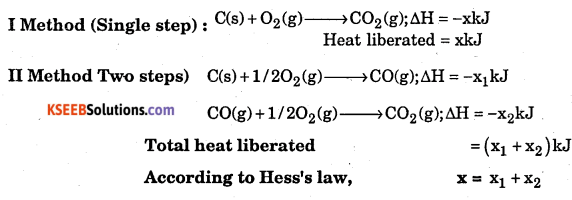Question 9.
Explain the meaning of thermochemical equation for the reaction.
2H2(g) + O2(g) → 2H2O(l) +136 kcal.
When two moles i.e., 4 grams of gaseous H2 reacts with 1 mole (32g) of gaseous O2 to give 2 moles of liquid water i.e., 36 gram of liquid water, 136 kcal of heat is liberated.Question 10.
Explain heat of neutralisation with an example.
Heat of neutralisation is the change in enthalpy produced when one gram equivalent weight of an acid is neutralised by one gram equivalent weight of a base in dilute solution.
Example : HCl(aq) + NaOH(aq) → NaCl(aq) + H2O;= ΔH – 57.3 kJ

Question 11.
With example explain the term ‘Heat of transition’.
Heat of transition is the charge in enthalpy produced when one mole of one allotropic form of an element is converted into another allotropic form.
Example : C(diamond) > C(graphite); ΔH = -X1 kJ When 1 mole of diamond is coverted into graphite x kJ of heat is liberated, -x kJ is the heat of transition in this case.

Question 12.
Define heat (enthalpy) of transition.
It is enthalpy change when one mole of an element is changed from one equilibrium to another.

Question 13.
What do you understand by the statements :
(i) enthalpy of formation of nitric oxide is +90.7 kJ.
(ii) enthalpy of combustion of carbon disulphide is =1065.2 kJ
(i) When 1 mole of NO(g) is formed from its elements N2(g). and O2(g), 90.7 kJ of heat is absorbed.
(ii) When 1 mole of CS2 (l) is completely burnt in O2,1065.2 kJ of heat is liberated.

Question 14.
Enthalpy of neutralisation of NH4OH with HC is 51.45 kJ. Calculate the enthalpy of ionization of ammonium hydroxide.
Enthalpy of ionzators of NH4OH = -51-41 – (57.3) = 5.8500 JQuestion 15.
Explain: “The enthalpy of formation of nitric oxide is +90.4 kJ”.
$$\frac { 1 }{ 2 }$$N2(g) + $$\frac { 1 }{ 2 }$$ O2(g) → ANO(g)ΔH = +90.4kJ When 1 mole of nitric oxide is formed from its elements 90.4 kJ of heat is absorbed. (Actually ΔH\+90.4kJ Error in the question)

Question 16.
Distinguish between exothermic and endothermic reactions.Question 17.
State and explain the first law of thermochemistry.
If enthalpy of formation of a compound is -x kJ, enthalpy of its decomposition intoits elements is + xkJ.
Example : C(s) + O2(g) → CO2; ΔH = -xkJ According to the law (Lavoisier & Laplae law) CO2 (g) → C(s) + O2 (g); ΔH = +x kJ

Question 18.
Which of the following has highest heat of combustion out of the following and why ?
(a) C2H6 (b) C2H4 (c) C2H2 (d) CH4
C2H6(g) will have highest heat of combustion because it has highest molecular weight
and second highest calorific value.
ΔHc = calorific value of kJ/g × mol. wt. = 52 × 30 = 1560 kJ/mol.Question 19.
What do you understand by state functions ? Neither q nor w is a state function but q + w is a state function ? Explain.
State function is a property whose value depends only uopon the state of system an is independent of the path, q and w are not state functions because they depend upon path. But q + w = AE which is a state function. AE does not depend upon path.

Question 20.
Acetic acid and hydrochloric acid react With KOH solution. The enthalpy of neutralization of acetic acid is -55.8 kJ mol-1 while that of hydrochloric acid is-57.3 kJ mol-1. Why?
It is because HCl is strong acid, it ionises completely in aqueous solution whereas acetic acid is weak acid which does not ionise completely and some energy is used in its complete ionisation.

Question 21.
Taking a specific example show that ΔStotal is a criterion for spontaneity of a change.
ΔG = -TΔStotal
If ΔStotal is +ve, ΔG will be -ve, reaction will be spontaneous.
If ΔStotal is -ve, ΔG will be +ve, reaction will be non-spontaneous,
ΔStotal = ΔSsyst + ΔSsurroundings
For example in case of freezing of water at 273 K, TAStotai is +0.08 J/K/mol whereas at 274 K it is -0.080 J/K/mol. Since the ΔS total is +ve at 273 K, freezing of water will take place. On the other hand at 274 K, the total entropy change is negative, the freezing will not occur.

Question 22.
What is meant by entropy driven reaction ? How can a reaction with positive changes of enthalpy and entropy be made entropy driven ?
Those reactions which are endothermic and entropy is increasing such that TΔS > ΔH are entropy driven reactions. If ΔS total is +ve, the reaction will be spontaneous. If ΔS is +ve and is small, but T is large, the reaction will be spontaneous.
A reaction which is endothermic, i.e., ΔH is +ve and ΔS is +ve, T must be large.
ΔG = ΔH – TΔS ; ΔG will be -ve if TΔS > ΔH, i.e., the reaction will be spontaneous at higher temperature because entropy will increase with increase in temperature which will make this reaction spontaneous.Question 23.
Justify the following statements:
(a) An exothermic reaction is always thermodynamically spontaneous.
(b) The entropy of a substance increases on going from liquid to vapour state at any temperature.
(a) It is false. Exothermic process are not always spontaneous. If ΔS = -ve and TΔS > ΔH, the process will be non spontaneous even if it is exothermic.
(b) The entropy of vapour is more than that of liquid therefore entropy increases during vaposisation.

Question 24.
Evaporation of water is an endothermic process but spontaneous. Explain.
It is because entropy increases -during this process because water vapour have more entropy than liquid water. ΔG become -ve because TΔS > ΔH.

Question 25.
What is meant by toral entropy change of a process ? Assuming the thermodynamic relationship ΔG = ΔH – TΔS, derive the relationship ΔG = TΔStotal for a system.
Total entropy change of a process if entropy change taking place in universe, i.e.
ΔSsystem + ΔSsurrounding = ΔSTotal
ΔSTotal = ΔSsys – $$\frac{q}{T}$$= ΔSSys – $$\frac{\Delta \mathrm{H}}{\mathrm{T}}$$
TΔSTotal = TASSys – ΔH [∵ ΔG = ΔH – TΔS]
TΔSTotal = -ΔG
ΔG = -TΔSTotal

Question 26.
At a certain temperature ‘T’ endothermic reaction A → B proceeds virtually to end. Determine the sign of ∆S for the reaction A → B and ∆G for the reverse reaction B →A.
∆S – +ve ∆H = +ve, ∆G = -ve the reaction proceeds virtually to end in reverse reaction B → A, ∆S = -ve, ∆G = +veQuestion 27.
Starting with the thermodynamics relationship G = H – TS, derive the following relationship ∆G = -T∆STotal
At initial state of the system Gi = Hi – T Si
At final state of the system Gf = Hf – TSf
∴ Change is free energy ∆G = Gf – Gi = (Hf – Hi) – T (St – Si)
∆G = ∆H – T∆S

Question 28.
Explain the help of example, the difference between bond dissociation energy and bond energy.
Bond disoociation energy is energy required to break 1 mole of bonds e.g.
H – H(g)) → 2H(g) ∆H = 436 kJ mol-1
Bond energy is energy released when 1 mole of bonds are formed example
2H →H2(g) ∆H = -436kJ mol-1
In diatomic molecule both bond dissociation energy and bond energy are equal in magnitude but opposite in sign.

Question 29.
What is meant by the free energy of a system ? What will be the direction of chemical reaction when (i) ∆G = 0 (ii) ∆G > 0 (iii) ∆G < 0 Answer: Free energy is defined as energy which can be converted into useful work (i) ∆G = 0, the reaction will be in equilibrium (ii) ∆G > 0, the reaction will not take place
(iii) ∆G < 0, the reaction will be spontaneous

Question 30.
Why most of the exothermic process (reactions) spontaneous ?
∆G = ∆H – ∆H – T∆S; For exothermic reactions ∆H is -ve. For a spontanec s process AG is to be -ve.
Thus decrease in enthalpy (-∆H) contributes significantly to the driving force (to make AG negative).

Question 31.
The enthalpy of combustion of sulphur is 297 kJ. Write the thermochemical equation for combustion of sulphur. What is the value of ΔfH of SO2 ?
Ans wer:
S(s) + O2(g) → SO2(g); ΔH = -297kJ and ΔfH of SO2 = -297 kJ mol-1.

Question 32.
What is the most important condition for a process to be reversible in . thermodynmics ?
The process should be carried out infinitesimally slowly of the driving force should be infinitesimally greater than the opposing force.Question 33.
Why absolute value of enthalpy cannot be determined ?
As H = E = +PV
Absolute value of E – the interned energy cannot be determined as it depends upon various factors whose value cannot be determined.
∴ Absolute value of H cannot be determined.

Question 34.
What are the applications of Hess’s Law of constant heat summation ?

• It helps to calculate the enthalpies of formation of those compounds which cannot be determined experiementally.
• It helps in determine the enthalpy of allotropic transformation like C (graphite) → C (diamond)
• It helps to calculate the enthalpy of hydration.

Question 35.
Two litres of an ideal gas at a pressure of 10 atm expands isothermally into a vaccum until its total volume is 10 litres. How much heat is absorbed and how much work is done in the expansion ?
We have q = – w : pext (10 – 2) = 0 × 8 = 0
No. work done; No heat, is absorbed.

Question 36.
Define (i) Molar enthalpy of fusion (ii) Molar enthalpy of vaporization ?
(i) The enthalpy change that accompanies melting of one mole of a solid substance at its melting point is called molar enthalpy of fusion.
(ii) Amount of heat required to vaporize one mole of a liquid at constant temperature and understand pressure (1 bar) is called molar enthalpy of vapourisaton.Question 37.
Define : (a) Enthalpy of atomization (b) Lattice enthalpy.
Enthalpy of atomization : It is defined as the enthalpy change accompyning the breaking of one mole of a substance completely into its atoms in the gas phase.
H2(g) → 2H(g); ∆aH° = 435.0kJ mol-1
Lattice enthalpy ; Lattice of an comp; the enthalpy change which occure when one mole of an ionic compound dissociates into its gaseous ionic state.
NaCl(s) → Na+ (g) + Cl (g); ∆H = +788 ks / mol

Question 38.
Explain Laplace- Lavoisier law.
The quantity of heat that must be supplied to decompose a compound into its elements is equal and opposite to the heat evolved when the same compound is formed from its elements.
C(s) + O2(g) → CO2(g) ∆H = -393.5 kJml-1
CO2(g) → C(s) + O2(g) ∆H = +393.5 kJml-1

Question 39.
If the enthalpy of combustion of diamond and graphite are – 395.4 mol-1 and -393.6kJ moh1. What is the enthalpy change for the C (graphite) → C (diamond) ?
C(diamond) + O2(g) → CO2(g) ∆H =-395.4 kJmol-1 …(1)
C(graphite) + O2(g) → CO2(g) ∆H = -393.6kJmol-1 … (2)
C(graphite) → C(diamond ) substracting (1) from (2), we get
C(graphite) → C(diamond) ∆H = -393.6 kJ – (-395.4 kJ), ∆H = +1.8 kJ mol-1

Question 40.
H2(g) + $$\frac { 1 }{ 2 }$$ O2(g) → H2O(g) ΔfH = -242kJ mol-1. Bond energy of H2 and O2 are 436 mol-1 and 500 mol-1 respectively. What is bond energy of O – H bond ?
H2(g) + $$\frac { 1 }{ 2 }$$ O2(g) → H2O(g); ΔfH° = -242kJ mol-1
H = Bond energy of reactions – Bond energy of products
ΔH = BH-H + $$\frac { 1 }{ 2 }$$BO – O + BO – H  ; 242kJ = 436 + $$\frac { 1 }{ 2 }$$ (500) – 2BO – H ⇔ -928kJ = -2BO – H; BO – H = $$\frac { 928 }{ 2 }$$ = 464kJ mol-1Question 41.
What is spontaneous process ? Give example.
Process which takes place itself, without any external aid under the given condition is called spontaneous process.
Example : Flow of heat from higher to lower temperature.

Question 42.
Define non-spontaneous process.
Process which does not takes place itself or on its own but with the help of external aid under the given condition is called non-spontaneous process.
Example : Flow of heat energy from lower to higher temperatures.

### 1st PUC Chemistry Thermodynamics Three Marks Questions and Answers

Question 1.
Classify the following processes as reversible or irreversible :
(i) Dissolution of sodium chloride
(ii) Evaporation of water at 373 K and 1 atm. pressure
(iii) Mixing of two gases by diffusion
(iv) Melting of ice without rise in temperature
(b) When an ideal gas expands is vaccum, there is neither absorption nor revalutions of what ? why ?
(a)(i) Irreversible, (ii) Irreversible, (iii) Irreversible, (iv) Reversible
(b) It is because no work is done,
i.e., w = 0 w = -pext × ∆V = 0 × ∆V = 0
In ∆U = q + w
q = 0 because gas chamber is insulated ∆U = 0 + 0 = 0Question 2.
Justify the following statements :
(a) Reaction with ∆G° < 0 always have an equilibrium constant greater thanl.
(b) Many thermodynamically feasible reaction do not occur under ordinary conditions.
(c) At low temperatures enthalpy change dominates the AG expression and at high temperature it is the entropy which dominates the value of AG.
(a) ∆G° = -2.303RT logK If K > 1, ∆G° will be less than zero because products formed are more than that of reactants, i.e., process is spontaneous in forward direction.
(b) It is because heat energy is required to overcome activation energy.
(c) ∆G = ∆H – T∆S, At low temperatures ∆H > T∆S whereas at high temperature T∆S >∆H
∴ ∆G decreases, i.e., becomes negative.

Question 3.
If for the reaction PbO2 → PbO, ΔG° < 0
And for the reaction SnO2 → SnO, ΔG° > 0
What are the most probable oxidation states of Pb and Sn ?
Oxidation states of the elements in the following compounds are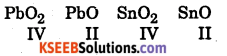Since PbO2 → PbO change is accompanied by decrease in free energy it is a spontaneous change hence Pb in +2 state is more stable than in +4 state.

Question 4.
(i) What is the value ΔrH° of the following reaction :
H+(aq) + OH(aq) →H2O(l).
(ii) Give an example in which enthalpy change is equal to internal energy ?
(i) ΔrH° = -57.1kJmol-1
(ii) H2(g) + I2(g) → 2HI(g) here ΔH = ΔU

Question 5.
Consider the reaction. A + B → C + D
(i) If the reaction is endothermic and spontaneous in the direction indicated, comment on the sign of ΔG and ΔS.
(ii) If the reaction is exothermic and spontaneous in the direction indicated, can you comment on the sign of G and S?
(iii) If the reactin is exothermic and spontaneous only in the direction opposite to the indicated, comment on the sign of AG and AS for the direction indicated in the equation.
(i) ΔG = -ve, ΔS = +ve
(ii) ΔG = -ve, ΔS = -ve (if ΔH > TΔS)
(iii) ΔG = ΔS = -ve

Question 6.
Predict in which of the following entropy increases / decreases.
i) Temperature of a crystalline solid is raised from 0 K to 115 K.
ii) 2NaHCO3 → Na2CO3(s) +CO2 (g) + H2O(g)
iii) H2(g) → 2H(g
(i) When temperature is raised, disorder in molecules increases and therefore entropy increases.
(iii) Reactant is a solid and hence has low entropy. Among the products there are two gases and one solid so products represent a condition of higher entropy.
(iv) Here 2 moles of H atoms have higher entropy than one mole of hydrogen molecule.

Question 7.
Which of the following is / are exothermic and which are endothermic ?
(i) Ca(g) → Ca2+(g) + 2e
(ii) O(g) + e → O (g)
(iii) N2-(g) + e → N3-(g)
(i) Endothermic (ionisation energy is required)
(ii) Exothermic (first electron affinity – energy is released)
(iii) Endothermic (higher electron affinities energies are required)### 1st PUC Chemistry Thermodynamics Numerical Problems and Answers

Question 1.
Calculate the heat of reaction of the following reaction :

C6H12O6(s) + 6O2(g) → 6CO2(g)+ 6H2O(g); ΔH = ?
C(graphite) + O2(g) → CO2(g); DH = -395.0 kJ … (1)
H2 (g) + $$\frac { 1 }{ 2 }$$O2(g) → H2O(l); DH = -269.5 kJ … (2)
6C(graphit.e) + 6H2(g) + O2(g) → C6H12O6(s); DH = -1169.8 kJ … (3)
Multiplying equation (1) and (2) each by 6 reversing (3), we get,
6C(graphite) + 6O2(g) → 6CO2(g); ΔH = -2370 kJ … (4)
6H2(g) + 3O2(g) → 6H2O(l); ΔH = -1616.4 kJ … (5)
C6H12O6(s) → 6C(graphite) + 6H2(g) + 3O2(g) ; ΔH = +1169-8 kJ …. (6)
Adding (4), (5) and (6), C6H12O6(s) + 6O2(g) → 6CO2(g) + 6H2O(g) ;
AH(C6H12O6) = -2370.0 – 1616.4 + 1169,8 = -2816.6 kJ

Question 2.
Calculate the heat of reaction of the following reaction :
CO2(g) + H2(g) → CO(g) + H2O(g)
Given that the ΔfH°CO(g) =-110.5kJ, ΔfH°CO2(g) =-393.8kJ,
ΔfH°H2O(g) = -241.8kJ respectively.
The required equation is CO2(g) + H2(g) → CO(g) + H2O(g)
ΔH – Σ ΔHf(products) — Σ ΔHf(reactants)
= ΔHf2CO(g) + ΔHf2H2O(g) – ΔHf2CO2(g) – ΔHf2H2(g)
= -110.5 kJ – 241.8 kJ – (-398.3 kJ) – 0 = -352.3 kJ + 393.8 kJ = 41.5 kJ.

Question 3.
1 m3 of C2H4 at STP is burnt in oxygen, according to the thermochemical
reaction: C2H4(g) + 3O2(g) → 2CO2(g) + 2H2O(l); ΔH = -1410 kJ mol-1
Assuming 70% efficiency, determine how much of useful heat is evolved in the reaction.
22.4 L of C2H4 at STP produces 1410 kJ of energy.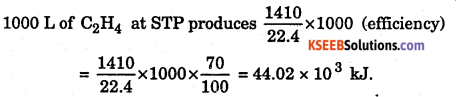Question 4.
With the help of thermochemical equation, calculate ΔfH° at 298 K for the following reactions:
C(graphite) + O2(g) → CO2(g) ; ΔfH° =-393.5kJ/mol
H2 (g) + $$\frac { 1 }{ 2 }$$O2(g) → H2O(l) ; ΔfH° = -285.8kJ/mol
CO2 (g) + 2H2O(l) → CH4 (g) + 2O2(g) ; ΔfH° = +890.3kJ/mol
C(graphite) + O2(g) → CO2(g) ; ΔfH° = -393.5kJ/mol …(1)
H2(g) + $$\frac { 1 }{ 2 }$$O2(g) → H2O(l) ; ΔfH° = -285.8kJ/mol …(2)
CO2(g) + 2H2O(l) → CH4(g) + 2O2(g) ΔfH° = +890.3kJ/mol …(3)
Here we want one mole of C(graphite) as reactant, so we write down equation (1) as such, we want two moles of H2(g) as reactant, so we multiply equation (2) by 2, we want

C(graphite) + O2 (g) → CO2(g) ; ΔfH° = -393.5kJ / mol …(1)
2H2(g) + O2(g) → 2H2O(1) ; ΔfH° = 2(-285.8kJ/mol) …(2)
CO2(g) + 2H2O(l) → CH4(g) + 2O2(g) ; ΔfH° =+890.3kJ/mol . … (3)
C(graphite) + 2H2(g) → CH4(g); ΔfH° =-74.8kJ/mol

Question 5.
The heat of combustion of C2H6 is -368.4 kcal. Calculate heat of combustion of C2H4, heat of combustion of H2 is 68.32 kcal mol-1.
C2H4(g) + H2(g) → C2H6(g) ; ΔH = -37.1 kcl
C2H4(g) + H2(g) → C2H6(g); ΔH = -37.1 k cal.
ΔHcC2H6 = -368.4 k cal, ΔHcC2H2 = ?; ΔHcH2(g) = -68.32 k cal.

ΔH  = ΣΔHc(reactanta) – ΣΔHc(products)
ΔH = ΔHcC2H4+ΔHcH2(g) – ΔHcC2H6(c)
-37.1k cal = ΔHcC2H4-68.32-(-368.4)
ΔHcC2H6 =-337.18 kcal.Question 6.
The following thermochemical equations represent combustion of ammonia and hydrogen:

4NH3(g) + 3O2(g) → 6H2O(l) + 2N2(g); ΔH = -1516 kJ
2H2(g) + O2(g) → 2H2O(l); ΔH = -572kJ
Calculate enthalpy of formation of ammonia.
Required equation is  $$\frac { 1 }{ 2 }$$N2(g) + $$\frac { 3 }{ 2 }$$H2(g) → NH3(g) ΔH = ?
4NH3(g) + 3O2(g) → 2N2(g) + 6H2O2(l) ΔH = -1516 kJ …(l)
2H2(g) + O2(g) → 2H2O(l) ΔH = -572 kJ …(2)
Reserving equation (1) and dividing by 4 we get:
$$\frac { 1 }{ 2 }$$N2(g) + $$\frac { 3 }{ 2 }$$2H2O2(l) → NH3(g)+ $$\frac { 3 }{ 4 }$$O2(g) ΔH = +379 kJ …(3)
Multiplying equation (2), by 3/4 we get,
$$\frac { 3 }{ 2 }$$H2(g) + $$\frac { 3 }{ 4 }$$O2 → $$\frac { 3 }{ 2 }$$H2O(l) ΔH = -572 × $$\frac { 3 }{ 4 }$$ = -429kJ
Adding (3) and (4) we get ,
$$\frac { 1 }{ 2 }$$N2(g) + $$\frac { 3 }{ 2 }$$H2(g) → NH3(g) ;ΔH = +379 – 429 = -50 kJ/mol

Question 7.
The equilibrium constant at 25° C for the process.
CO3+(aq) + 6NH3(aq) ⇌ [CO(NH3)6]3(aq) is. 2.5 × 106. Calculate the value of ΔG° at 25° C. (R = 8.314 JK1 mol-1). In which direction is the reaction spontaneous under standard conditions ?
ΔG° =-2.303 RT log K .
= -2.303 × 3.134 × 298 log (2.5 x 106)
= -5705.8 [0.3980 + 6.0000] = -5705.8 × 6.3980 = -36.505 k/mol.
The reaction is spontaneous in forward direction under standard conditions.

Question 8.
What is the value of equilibrium constant for the following reaction at 400 E?
2NOCl(g) ⇌ 2NO(g) + Cl2(g)
ΔH0 = 77.5kJmol-1, R = 8.3124 J Mol-1K-1, AS = 135 J K-1mol-1
ΔG = ΔH – TΔS
ΔG = 77.5 × 1000 J – 400 K × 135 J K-1 = 77500 J – 54000 J = 23500 J
ΔG = -2.303 RT log K
23500 J = -2.303 × 8.314 × 400 K log K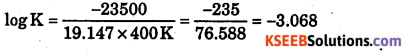Log k =- 3.068 k = antilog(-3.008)
= Antilog (0.932-4) = Antilog (0.93) × 10-4 = 8055 × 10-4

Question 9.
The standard free energy change for a reaction is -212.3 kJ mol-1. If the enthalpy of the reaction is -216.7 kJ mol-1, Calculate the entropy change for the reaction.
ΔG° = -212.3 kJ mol-1, ΔH0 = -216.7 kJ mol-1, ΔS° = ?
T = 298 K (Because standard free energy is measured at 298 K)
ΔG° = -212.3 kJ mol-1, ΔH° = 216.7 kJ mol-1, ΔS° = ?
-212.3 kJ = 216.7 kJ – 298 K × ΔS°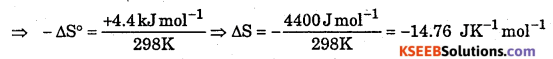Question 10.
Calculate the standard free energy change AG° for the reaction.
2HgO(s) → 2Hg(l) + O2(g)
ΔH° = OlkJmol-1 at 298 K, S°(Hg0) = 72.0JK-1 mol-1
ΔS = 2S°Hg + S°(O2) – 25°Hgo
= (2 × 77.4 + 205 – 2 × 72.0) JK -1mol-1 = 215.8 jK-1 mol-1
ΔG° = ΔrH° – TΔrQuestion 11.
From the data given below at 298 K for the reaction :
CH4(g) + 2O2(g) → CO2(g) + 2H2O(l)
Calculate the enthalpy of formation of CH4(g) at 298 K.
Enthalpy of reaction is = -89.5 kJ
Enthalpy of formation of CO2(g) – -393kJ mol-1
Enthalpy of formation of H2O(l ) = -286.0 kJ mol-1
ΔH = AHfCO2(g) + 2ΔHfH2O(l) – ΔHfCH4(g) – ΔHf;O2(g)
-890.5 kJ = -393.5 kJ +2 × -286 kJ – Δ HfCH4(g) – 0
ΔHfCH4 – 75.0kJ

Question 12.
Calculate the free energy change when 1 mole of NaCl is dissolved in water at 298 K. (Given : Lattice energy of NaCl = – 777.8 kJ mol-1), Hydration energy
= -774.1 kJ mol-1 and ΔS = 0.043 kJK-1 mol-1 at 298 K.)
ΔH = Hydration energy – Lattice energy
ΔH = -774.1 kJ mol-1 (-777.8 kJ mol-1) = 3.7 kJ mol-1
ΔG = ΔH – TΔS = +3.7 kJ- 298 × 0.043 kJ = +3.7 kJ- 12.81 kJ
ΔG = -9.11 kJ moHQuestion 13.
For the equilibrium, PCl5 (g) ⇌ PCl3 (g) + Cl2 (g) at 298 K, K = 1.8 × 10 -7.
Calculate ΔG° for the reaction (R = 8.314JK 1mol 1)
PCl5(g) ⇌ PCl2(g) + Cl2(g), K = 1.8 × 10-7
ΔG° = -2.303RTlogK = -2.303 × 8.314JK-1mol-1 × 298K × log(1.8 × 10-7)
= -2.303 × 8.314 × 298[log 1.8 + logl0-7 ] = -19.147 × 298 [0.2553 – 7.000]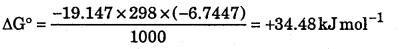Question 14.
Calculate the entropy change involved in conversation of 1 mole of water at 373 K to vapours at the same temperature. Latent heat of vaporation of water = 2.257 kJ g1.
k = 1.8 × 10-7
ΔH = 2.257 × 18kJ= 40.626kJ mol-1Question 15.
Calculate ΔHf of HCl if bond energy of H – H bond is 437 kJ Cl – Cl bond is 244, and H – C is 433 kJ mol1.
$$\frac { 1 }{ 2 }$$H2(g) + $$\frac { 1 }{ 2 }$$ cl2(g) > HCl(g)
ΔH = $$\frac { 1 }{ 2 }$$BH – H + $$\frac { 1 }{ 2 }$$BO – O = $$\frac { 1 }{ 2 }$$ × 437 + $$\frac { 1 }{ 2 }$$ × 244 -433
= 218.5 kJ + 122 kJ – 433 kJ = -92.5 kJ mol-1

Question 16.
Calculate bond energy of C – H bond if ΔHc of CH4 is -891 kJ, ΔHc of C (s) is – 394 kJ, ΔHc of H2(g) is -286 kJ, heat of sublimation of C(s) is 717 kJ, heat of dissociation of H2 is 436 kJ.
CH4(g) + 2O2(g) → C02(g) + 2H2O(l) ; ΔH = -891 kJ
C(s) + O2(g) → CO2(g) ;ΔH = -394 kJ
H2(g) + $$\frac { 1 }{ 2 }$$ O2(g) → H2O(l) ; ΔH = -286 kJ
C(s) → C(g) ;ΔH = +717 kJ
H2(g) → 2H(g);Δ H = +436 kJ
Target equation is CH4 +4H(g)
Reversing (ii),Multiply (iii) by 2 and reverse, Multiply eqn. (v) 2 and then adding all together we get:
CH4 → C(g) + 4H(g) ΔH = -891kJ + 394kJ + 575kJ + 717kJ + 872kJ
ΔH = 1664 kJ
Energy required to break 4(C – H) bond = 1664 kJ
Energy required to break one (C – H) bond =\frac{1664}{4} = 416 kJ mol-1Question 17.
What would be heat released when :
(i) 0.25 mole of HCl in solution is neutralized 0.25 mole of NaOH solution ?
(ii) 0.5 mole of HNO3 in solution is mixed with 0.2 mole of KOH solution ?
(iii) 200 cm3 of 0.2 M HCl is mixed with 300 cm3 of 0.1 M NaOH solution ?
(iv) 400 cm3 of 0.2 M H2SO4 is mixed with 300 cm3 of 0.1 M NaOH solution ?
(i) H+(0.25mole) + OH(0.25mole) → H2O(0.25mole)
Heat released = 0.25 × 57.1 = 14.3 kJ
(ii) 0.5 mole HNO3 (aq) + 0.2 mole KOH(aq)
The net reaction is (0.2 mole HNO3 reacts with only 0.2 mole KOH)
0.2 mole is limiting reagent.
0.2 mole of H+ + 0.2 mole of OH → 0.2 mole of H2O
0.3 mole of H+ will remain unreacted.
Heat evolved = 0.2 × 57.1 = 11.4 kJ

(iii)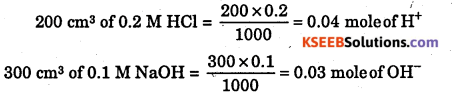0.03 mole of OH is limiting reactant
Heat evolved = 0.03 × 57.1 = 1.71 kJ

(iv)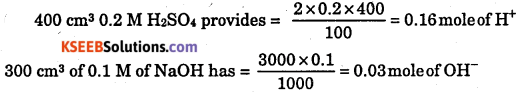0.03 is limiting reagent Heat evolved = 0.03 × 57.1 = 1.7 kJ

(v) Mass of solution = 200 + 300 = 500 g in (iii) part (Assuming d = 1 g cm 3)
q = m × c × T 1.71 × 1000 J = 500 g × 4.18 × T
T = 0.82 K For (iv), T = $$\frac{1.7 \times 1000}{700 \times 4.18}$$ = 0.58Kerror: Content is protected !!